# Biconvex Lens - Definition, Properties, Uses

## Introduction

Biconvex lens we see in a magnifying glass.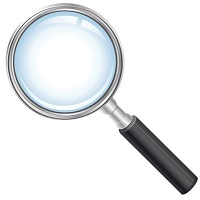You must have used a magnifying glass to read text that was too small. A magnifying glass is also the icon that is used in mobiles and apps when the “search” function is involved.

Further, if people in your family wear glasses, you must have noticed that everyone wears a different type. For some people, the glasses are thin while for others, they are much thicker.

Another interesting thing you must have noticed is that near-vision glasses show things bigger than they are, while far-vision glasses do the opposite.

This happens due to the different types of lenses utilized in different scenarios. Depending on the shape, lenses are classified into various types, some of which include convex, concave, biconvex, biconcave, etc. In this article, we will discuss biconvex lenses in detail.

## Biconvex Lenses- Definition and Meaning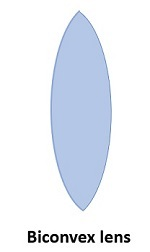A lens is said to be biconvex when both of its surfaces are convex, i.e., they are curved outwards. The two sides can be perfectly symmetrical, but that is not a necessity. The focal length on either side can be different. In the diagram below, you can see a symmetrical biconvex lens. When both sides have equal radii of curvature, the lens is said to be equiconvex.

As you can see, a biconvex lens is a converging lens. This means that when light rays parallel to the principal axis are incident on it, they tend to converge at its focus.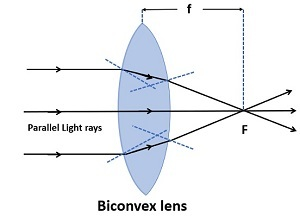The focal length of a biconvex lens is taken to be positive by convention.

## Biconvex Lenses- Properties

A few important properties of the biconvex lenses are

• A biconvex lens converges light that is incident on its surface and is said to possess a positive focal length.

• Biconvex lenses can form real as well as virtual images.

• Objects viewed through a biconvex lens appear larger than they are under certain scenarios.

• Objects viewed via biconvex lenses are closer than they appear to be.

### Focal Point of Biconvex Lenses

Take a look at the diagram below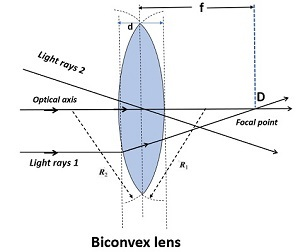The light ray marked “Light ray 1” is incident parallel to the principal or optic axis of the lens and it meets the optic axis at a point D. Interestingly, this would happen regardless of the position of the light ray. Any ray that is parallel to the principal axis will refract and meet the principal axis at point D. The point D, where such rays of light converge is known as the focal point of the lens.

By convention, the focal point of the biconvex lens is on the right since light is assumed to be incident from the left. Further, if the lens is symmetrical or equiconvex, the distance of the focal point from the optical center would be the same on either side of the lens.

## Biconvex Lens Formula

To derive the formula for a biconvex lens, we start with the lens equation, i.e.,

$$\mathrm{\frac{1}{v}-\frac{1}{u}=\frac{1}{f}}$$

Further, if the light is incident on a spherical surface of a medium with refractive index $\mathrm{n_2}$ and radius R, then the relation between image distance and object distance is given by:

$$\mathrm{\frac{n_2}{v}-\frac{n_1}{u}=\frac{n_2-n_1}{R}}$$

Where $\mathrm{n_1}$ is the refractive index of the first medium.

Now, consider the biconvex lens (shaded in purple) given in the figure below, made by the common parts of two spheres. Its refractive index is $\mathrm{n_2}$, while it is placed in a medium of refractive index $\mathrm{n_1}$. Further, the radii of curvature of surfaces on the left and right of the lens are $\mathrm{R_1}$ and $\mathrm{R_2}$, respectively.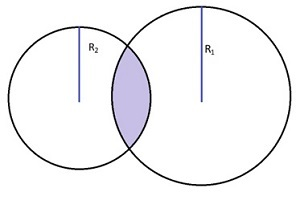Using the relations given above, we have, for the left surface −

$$\mathrm{\frac{n_2}{v_1}-\frac{n_1}{u}=\frac{n_2-n_1}{R_1}}$$

Similarly, for the surface on the right, the equation reads −

$$\mathrm{\frac{n_1}{v}-\frac{n_2}{v_1}=\frac{n_1-n_2}{R_2}}$$

Adding these equations, we see that $\mathrm{\frac{n_2}{v_1}}$ cancels out, and we are left with have −

$$\mathrm{n_1(\frac{1}{v}-\frac{1}{u})=(n_2-n_1)(\frac{1}{R_1}-\frac{1}{R_2})}$$

Let $\mathrm{\mu=\frac{n_2}{n_1}}$

Further, let f represent the focal length of the above lens. Finally, for a biconvex lens, $\mathrm{R_1}$ is positive, while $\mathrm{R_2}$ is negative. Thus, with the sign convention applied, the biconvex formula reads as below −

$$\mathrm{\frac{1}{f}=(\mu-1)(\frac{1}{R_1}+\frac{1}{R_2})}$$

## Biconvex Lenses- Uses & Applications

Biconvex lenses find applications in the following scenarios −

• Since they produce larger images, they are used as magnifying glasses.

• Objective lenses of various optical apparatuses like microscopes and telescopes are made via biconvex lenses.

• The human eye is also a biconvex lens.

• Biconvex lenses are also used to make vision correction glasses.

• Photographic sensors and cameras utilize biconvex lenses for focus purposes.

## How to Make Biconvex Lenses?

While you cannot manufacture high-quality glass without the use of specialized equipment, you can still fabricate a DIY biconvex lens using some plastic bottles. Follow the steps given below.

All you need is a transparent plastic bottle, some hot glue, and some water.

• Take a plastic bottle, preferably one whose mouth is approximately 2.5 cm in diameter. Make sure that the bottle you pick is clean and smooth.

• Use the bottle’s cap to draw two circles on the surface of the bottle.

• Cut out the circles you have drawn.

• Use a hot glue gun to seal the edges of the two circles together, but make sure that you leave some space between them. Further, leave a small opening when you join the circles.

• Now, when you dip these two conjoined pieces in water, the water will flow in via the opening you left out and fill the gap.

• You now have a makeshift biconvex lens. The water serves as the material of the lens, and it is contained inside the circular plastic pieces you cut out.

To try out this homemade lens, you can try reading some small text with it. As you will notice, a biconvex lens makes the text appear larger.

## Conclusion

Lenses are used almost everywhere in the scientific world and their application is not limited to physics. Indeed, almost all branches of science use lenses in one way or another.

Depending on their shape, lenses are categorized into various types, of which biconvex lenses are one. Biconvex lenses have two convex surfaces and thus, they converge light rays that are incident on them. For that reason, they are also known as converging lenses. The focal point of a biconvex lens is on the right and its distance from the optical center is equal to the focal length of the lens. Light rays that are incident parallel to the optic axis converge at the focal point.

With the sign convention already applied, the lens maker formula for a biconvex lens reads −

$$\mathrm{\frac{1}{f}=(\mu-1)(\frac{1}{R_1}+\frac{1}{R_2})}$$

Biconvex lenses have a wide array of applications, including magnifying glasses, objective lenses, focussing systems, etc. A makeshift biconvex lens may be made at home using some hot glue, a transparent plastic bottle, and some water.

## FAQs

Q1. Is the lens maker formula the same for all kinds of lenses?

Ans. The form of the lens maker formula does not change. However, before applying the formula, the sign convention must be considered. For example, for a concave lens, the radius of curvature is negative by convention. Thus, the lens maker formula varies slightly for each kind of lens.

Q2. How can biconvex lenses produce both real and virtual images?

Ans. The type of image formed by a biconvex lens depends on the position of the object. It may be real or virtual. For example, if the object is very far away, its image will be real. However, if placed close to the lens, we will have a virtual image instead.

Q3. What is the main point of difference between a prism and a lens?

Ans. A lens and a prism both refract light. However, prisms are unable to focus the light rays on a particular spot and thus, cannot form an image

Q4. How are biconvex lenses used in vision correction glasses?

Ans. People with farsightedness or hypermetropia have biconvex or convex lenses in their glasses.

Q5. Is the lens maker formula given above valid for any lens?

Ans. The lens maker formula we derived is for a thin lens. If the lens had a non-negligible thickness, the formula would be changed.

Updated on: 12-Apr-2023

255 Views